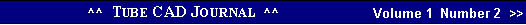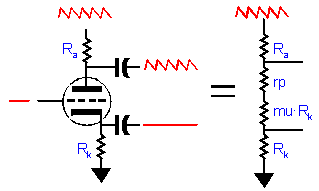But didn't we want the input signal to be the input of the phase splitter, not the power supply? In fact, both can be. The secret is to design a PSRR figure of -6 dB into the gain stage, so that the input signal is amplified and 50% of the power supply noise is added to the mix. The Grounded Cathode amplifier can easily be made to meet this requirement by varying the value of its unbypassed cathode resistor.    Here is a quick review of the voltage division preformed in a Grounded Cathode amplifier: the rp defines the bottom leg of the voltage divider; the plate resistor, the top leg. If the rp equals the plate resistor, the division is 50%. If an unbypassed cathode resistor is added, the effective rp increases by the mu plus one times the cathode resistor value:        rp' = rp + (mu + 1)Rk  ,and since we want rp' to equal the plate resistor value:        Rk  = (Rplate - rp) / (mu + 1) .    The key variable here is "rp." Triodes have it and pentodes barely do and transistors and MOSFETs don't at all. It is the resistance to AC signals offered by the plate. Pull the B+ voltage up and the triode fights back by increasing its current conduction; push the B+ voltage down and the triode fights back by decreasing its current conduction. It is this bite or grip on the plate voltage that gives the triode an edge over every other amplifying device. It constitutes a direct and instant feedback system that other devices must match through involved and circuitous global feedback loops.   Back to the circuit       So here is our line up so far: one grounded cathode amplifier without cathode resistor bypassing, one Split-Load phase splitter. Actually, we cannot easily DC couple the first stage into the second, because of the DC voltage levels. What we find when we match the tube's rp to the plate resistor value is that we have also matched the effective DC resistance: the DC voltage division also equals 50%. This means half the B+ voltage would appear at the cathode of the Split-Load phase splitter and half of voltage would appear at the plate, leaving 0 volts across the triode. If a true, two-resistor voltage divider were placed between the plate of the Grounded Cathode amplifier and ground and its output DC connected to the next stage's grid, the voltage at the Split-Load phase splitter would equal one fourth of the B+ voltage, and the voltage at its plate would equal three quarters of the B+ voltage. That would leave one half of the B+ voltage for the triode; the same ratio as the first stage, in fact. Because the voltage division is meant to be performed solely on the DC component of the plate voltage, the top resistor must be capacitor bypassed and the resistor values can be extremely large, 1 to 10 meg.
 Split-Load Phase Splitter    No, the better approach is to use a circuit known for its poorly balanced PSRR figures: the Split-Load phase splitter. This circuit has a very good PSRR figure at its cathode connection and a very poor to nonexistent PSRR figure at its plate. Its balance, however, is superb. The paradox disappears once we realize that we can use its poorly balanced PSRR figures to our advantage.Thought Experiment     Place a 50% voltage divider between B+ and ground; feed the output from this divider into the grid of a Split-Load phase splitter; estimate the amount and phase of the power supply noise at the phase splitter's outputs. We said earlier that the cathode connection had a very good PSRR figure, which means that the 50% of the power supply noise coming out of the voltage divider will be transferred entirely to the cathode and at the other end, 100% of the power supply noise will find its way to the plate. But as the Split-Load's plate connection will invert the signal at its grid, the one half division of noise will be inverted and added to the full power supply noise at the plate. 100% minus 50% equals 50%. Thus, the cathode and plate will share the same amount of power supply noise.Pg. 6